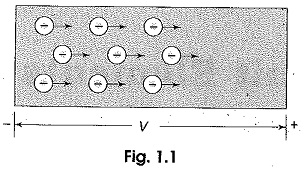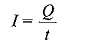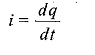# What is Current

## What is Current:

What is Current – There are free electrons available in all semiconductive and conductive materials. These free electrons move at random in all directions within the structure in the absence of external pressure or voltage. If a certain amount of voltage is applied across the material, all the free electrons move in one direction depending on the polarity of the applied voltage, as shown in Fig. 1.1.This movement of electrons from one end of the material to the other end constitutes an electric current, denoted by either I or i.The conventional direction of current flow is opposite to the flow of — ve charges, i.e. the electrons.

Current is defined as the rate of flow of electrons in a conductive or semiconductive material. It is measured by the number of electrons that flow past a point in unit time. Expressed mathematically,where I is the current, Q is the charge of electrons, and t is the time, orwhere dq is the small change in charge, and dt is the small change in time.

In practice, the unit ampere is used to measure current, denoted by A. One ampere is equal to one coulomb per second. One coulomb is the charge carried by 6.25 x 1018 electrons. For example, an ordinary 80 W domestic ceiling fan on 230 V supply takes a current of approximately 0.35 A. This means that electricity is passing through the fan at the rate of 0.35 coulomb every second, i.e. 2.187 x 1018 electrons are passing through the fan in every second; or simply, the current is 0.35 A.

Updated: September 29, 2017 — 8:26 pm